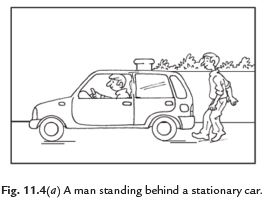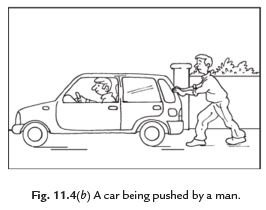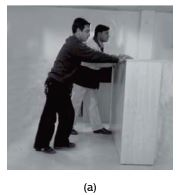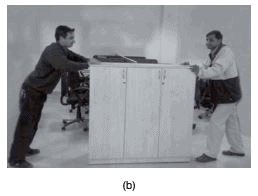Courses

# Short Answers - Force and Pressure, Science, Class 8 Class 8 Notes | EduRev

## Class 8 : Short Answers - Force and Pressure, Science, Class 8 Class 8 Notes | EduRev

The document Short Answers - Force and Pressure, Science, Class 8 Class 8 Notes | EduRev is a part of the Class 8 Course Class 8 Science by VP Classes.
All you need of Class 8 at this link: Class 8

Q. 1. What is a force? Explain with the help of some examples.
Ans.
Force is a pull or push of the objects. The action like, pushing, pulling, picking, hitting, lifting, running and bending are the examples of force. Moving or stopping of a body, changing shape and direction of motion of objects are various actions which show force in play.

Q. 2. How do we feel force in our daily life?
Ans.
Various big or small actions make us feel the force. We hit or catch many objects in our daily life. We see that a moving ball stops on its own. The ball changes the direction of its motion when it is hit with a bat. We make lassi etc. by churning of curd. These are many actions which help us to feel that a force is exerted.

Q. 3. Explain that forces are due to an interaction between objects.
Ans.
Imagine, a man is standing behind a stationary car. The car does not move due to his presence.Now allow the man to push the car, it means he applies a force on the car. The car may begin to move in the direction of the applied force. Note that the man has to push the car to make it move. This example shows that at least two objects must interact with each other for a force to come into play.

Q. 4. What happens when (i) Two forces are exerted in same direction?
(ii) Two forces are exerted in opposite directions?
Ans.
(i) When two forces are exerted in same direction on an object, then the forces are added and action becomes easy.
(ii) When two forces act in the opposite directions on an object, the net force acting on it is the difference between the two forces.Fig. 11.5 Two friends pushing a heavy load (a) in the same direction, (b) in opposite directions.

Q. 5. What are the two factors on which effect of force depends?
Ans.
There are following two factors on which the effect of force depends:

(i) Magnitude: The strength of force is usually expressed by its magnitude.

(ii) Direction of force: We have also to specify the direction of force in which it acts.
If the direction or magnitude of force changes, its effect also changes.

Q. 6. What are the effects of force?
Ans.
A force changes or try to change the
(i) Speed of a moving body.
(ii) Direction of motion of a body.
(iii) Shape of a body.

Q. 7. What are states of motion?
Ans.
An  object can be in two positions, one is at rest or other in motion ; both are its states of motion. Any change in this position (rest or motion) can be called the change in the state of motion. The state of motion of an object is described by its speed and the direction of motion.

Q. 8. How can a force change the states of motion?
Ans.
There are two states of an object:

(i) Rest and (ii) Motion.
When a force is applied on a body then it can move. In the same way a force can change the direction of a moving object as well as its speed. A force can stop a moving object.
If the force is applied in the direction of motion at an object, then its speed is increased. If the force acts in opposite direction, then the speed of the object is decreased.

Q. 9. What is the effect of force on the shape of an object?
Ans.
A force can change or try to change the shape of an object. When a force is applied on an object then change in shape takes place. It may be smaller or greater. At last we can say that the application of force on an object may change its shape.

Q. 10. Explain contact and non-contact forces.
Ans.
Contact forces: The forces which come into play only when two objects come in contact with each other are called contact forces. Muscular force and force of friction are the examples of contact forces.
Non-contact forces: The forces which come into play without any contact of objects with one another are called non-contact forces. Electrostatic and magnetic forces are the examples of non-contact forces.

Q . 11. What is muscular force? Why is it called contact force?
Ans.
The force resulting due to the action of muscles is known as the muscular force. Muscular force is called contact force because it comes in action only when two bodies come in contact with each other.

Q . 12. What do you understand by the force of friction?
Ans.
The force which always acts on all the moving objects and whose direction is always opposite to the direction of motion is called force of friction. Since the force of friction arises due to contact between surfaces, it is also called contact force.

Q . 13. What is electrostatic force? Why is it called non-contact force?
Ans.
The force exerted by a charged body on another charged or uncharged body is called electrostatic force. This force comes into play even when the bodies are not in contact, so it is called non-contact force.

Q . 14. Explain force of gravity.
Ans.
Objects or the things that fall towards the earth because the earth pulls them. This force is called force of gravity or the force due to gravitation. This is also called just gravity. It is an attractive force. This force acts on all objects.

Q . 15. What is pressure? What happens to the pressure when area on which it is applied increases?
Ans.
The force acting on a unit area of a surface is called pressure.
Pressure = Force/Area on which it acts.
The pressure is inversely proportional to the area on which force is applied. As the area on which force is applied is increased, the pressure decreases. We can say that pressure increases with decrease in area.

Q . 16. We observe that the wheels of buses and trucks are heavier than the wheels of cars or scooters. Why?
Ans.
The buses and trucks are heavy and require to exert less pressure. We know that pressure decreases as area of contact increases, so the tyres are broadened so that they exert less pressure on earth and move easily. If they exert more pressure, they will sink in earth. The scooters and cars have less wide wheels because they need more pressure for gripping.

Q . 17. What is atmospheric pressure?
Ans.
The envelop of air around the earth is known as atmosphere. The atmospheric air extends up to many kilometres above the surface of the earth. The pressure exerted by the air is called atmospheric pressure.

Q . 18. If the area of your head is 15 cm × 15 cm, how much air (in weight) would you carry on your head?
Ans.
The weight of air column of the height of the atmosphere and area 15 cm × 15 cm is equal to  the  weight  of  an  object  of  mass  225  kg  (2250  N).  We  have  air  inside  our  body,  so  we  are  not crushed under this weight and pressure of air is equal at both the sides.

Offer running on EduRev: Apply code STAYHOME200 to get INR 200 off on our premium plan EduRev Infinity!

90 docs|18 tests

,

,

,

,

,

,

,

,

,

,

,

,

,

,

,

,

,

,

,

,

,

,

,

,

,

,

,

;